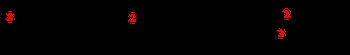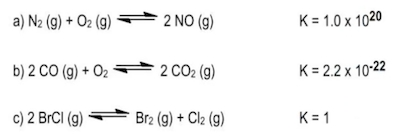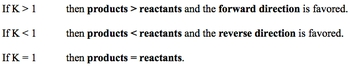Start typing, then use the up and down arrows to select an option from the list.# GOB Chemistry

Learn the toughest concepts covered in your GOB - General, Organic, and Biological Chemistry class with step-by-step video tutorials and practice problems.

Energy, Rate and Equilibrium

# The Equilibrium Constant

Equilibrium Constant K is associated with any reaction at equilibrium. Its numerical value determines if reactants or products are more greatly favored within a reaction.

## The Equilibrium Constant K

1

#### concept

The Equilibrium Constant Concept 12m
Play a video:
Hey, guys, In this brand new video, we're gonna take a look at the equilibrium. Constant. Now we're going to say that the variable we usedto illustrate our equilibrium. Constant is the variable K. We're gonna say K is a number equal to the ratio of products to react. It's at a given temperature. Why do I say at a given temperature? Because we're gonna say that temperature directly effects are equilibrium, constant K increasing the temperature will increase our K value. Decreasing our temperature will decrease r k value. Now we're gonna say it's a ratio of products to react in. So we're gonna say here that K equals products, overreact INTs. Now we're gonna say that K is important because it it's magnitude tells us how far to the left or to the right are. Chemical reaction is at a given temperature. So we're gonna say if K is greater than one than products, our favorite overreact. It's and think about it. This makes sense because we said K equals products. Overreactions. So let's say our products are 10 on our reactant are one k equals 10 definitely greater than one. We're gonna say when products are favorite over our reactant. That means we're making more products. How do we make more products by going in the forward direction so the four direction would be favored now in the opposite way if K is less than one? So let's say we have. In this case, products are one, but our reactions air 10 we have a K less than one. So in this case, if K is less than one than react INTs our favorite over products, which means our reaction is heading in the reverse direction so the reverse direction would be favored. So I just remember these two differences when K is greater than one when K is less than one Now, what if we say Kay was equal toe one? We know that this is products, overreact INTs. So that means that our products and reactant would have to be equal. Let's say they're both 10. 10/10 is one. So when K equals one, we're gonna say both are reacting in our product. Amounts are equal to one another. Now we're gonna say the equilibrium constant K takes into account all the states of matter except to it doesn't look at solids, and it doesn't look at liquids. It ignores those two states for matter

The Equilibrium Constant K is a ratio of products to reactants. It only deals with gaseous or aqueous compounds.2

#### example

The Equilibrium Constant Example 13m
Play a video:
so it looks at it only cares about really gasses and also acquis a quiz Just mean that the solvent is water. So a compound is dissolved in water. It would be a quiz. Now, knowing this, let's take a look at at the following example it says right, the equilibrium expression for the following reaction. Here we have to end 205 Acquis gives us four and 02 acquis plus O two. Acquis. It's important to look at the phases because remember, if it's a solid or liquid, we ignore it. Now we're gonna say here K equals products, overreact INTs So we're gonna have our products is n 02 on. Remember, you have to use the coefficients in these calculations. The number in front of No. Two is a four, so that four will become the power. So it's gonna be no to to the fore times 0202 just has a coefficient of one in front of it, which we don't have to show divided by and 205 again, the coefficient in front of end 12 5 is too. So that becomes a power. So we would say that this represents our equilibrium, expression or are equilibrium equation Same thing. Equilibrium, expression, equilibrium, equation. Now that we've seen this one, let's look at B okay, for be we're gonna say here, we're gonna ignore this compound because it's a solid and we're gonna ignore this compound because it's a solid here. We're gonna say K equals products. Overreacting. It's like before, but here's the thing we're gonna say we don't have any products available, but you have to put something on top. We're gonna say it's equal toe one solids and liquids are ignored, and in place of them, we're gonna put one. We're going to say this because things such as pressure don't really affect solids and liquids like they do gasses and acquis compounds. That's why they're equal toe one because their numbers being held constant. Okay, so we're gonna replace solids and liquids with the number one on on the bottom. We'd have 02 now differently your professor could give you this is we know there's a one here, so this could also be or in verse one. So be aware of both types of answers. Both are correct. Both are saying the same thing in verse. One just means one over whatever it ISS for the next one. Again, we ignore solids. The only thing that we don't ignore is this gas. So here K equals products. Overreact INTs, so just equal x e cute because of the three over one. But we don't need to put the one because anything over one is the same exact thing. So that would be our equilibrium expression. So it was pretty simple. But remember the differences in B. N. C. We ignore the solids and liquids. Now that you've seen this, I want you guys to attend to answer a question that follows this one. The practice one. Here. I want you to tell me who's greater in amount is a products or is a reactant from that. You have to remember, what do we say about K when it's greater than one who's favored when it's less than one who's favored when it's equal toe? One who's favored remembering that will be a great way for you to approach this problem. Good luck, guys.
3
Problem

State which is greater in amount:reactants or products, based on the given equilibrium constant, K.4
Problem

The decomposition of nitrogen monoxide can be achieved under high temperatures to create the products of nitrogen and oxygen gas.

6 NO(aq) ⇌ 3 N2(aq) + 3 O2(aq)

a) What is the equilibrium equation for the reaction above?

b) What is the equilibrium expression for the reverse reaction.

5
Problem

The equilibrium constant, K, for 2 NO (g) + O2 (g) ⇌ 2 NO2 (g) is 6.9 x 102

What is the [NO] in an equilibrium mixture of gaseous NO, O2, and NO2 at 500 K that contains 1.5 x 10 –2 M O2 and 4.3 x 10 –3 M NO2?